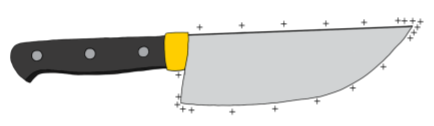$$\require{cancel}$$

18.4: Electric field and potential at the surface of a conductor

If we consider a conducting sphere of radius, $$R$$, with charge, $$+Q$$, the electric field at the surface of the sphere is given by: \begin{aligned} E=k\frac{Q}{R^2}\end{aligned} as we found in the Chapter 17. If we define electric potential to be zero at infinity, then the electric potential at the surface of the sphere is given by: \begin{aligned} V=k\frac{Q}{R}\end{aligned} In particular, the electric field at the surface of the sphere is related to the electric potential at its surface by: \begin{aligned} E=\frac{V}{R}\end{aligned} Thus, if two spheres are at the same electric potential, the one with the smaller radius will have a stronger electric field at its surface.

Because a conducting sphere is symmetric, the charges will distribute themselves symmetrically around the whole outer surface of the sphere. The charge per unit area, $$\sigma$$, at the surface of the sphere is thus given by: \begin{aligned} \sigma &= \frac{Q}{4\pi R^2}\end{aligned} The charge density can be related to the electric field at the surface of the sphere: \begin{aligned} E&=k\frac{Q}{R^2}=k\frac{4\pi R^2\sigma}{R^2}=4\pi\sigma k=\frac{\sigma}{\epsilon_0}\end{aligned} where in the last equality, we used $$k$$ with $$\epsilon_0$$ and confirmed the general result from Section 17.3, where we determined the electric field near a conductor with surface charge, $$\sigma$$.

Consider a sphere of radius, $$R_1$$, that carries total charge, $$+Q$$. A neutral second, smaller, conducting sphere, of radius $$R_2$$ is then connected to the first sphere, using a conducting wire, as in Figure $$\PageIndex{1}$$.Figure $$\PageIndex{1}$$: Two conducting spheres are connected by a conducting wire. The charge $$Q$$ that was originally on the larger sphere distributes itself onto the two spheres.

Because the charges on the large sphere can move around freely, some of them will move to the smaller sphere. Very quickly, the charges will stop moving and the spheres of radius, $$R_1$$ and $$R_2$$, will end up carrying charges, $$Q_1$$ and $$Q_2$$, respectively (we assume that the wire is small enough that negligible amounts of charge are distributed on the wire). Since the two conducting spheres are connected by a conductor, they form an equipotential, and are thus at the same voltage, $$V$$, relative to infinity. Since the two spheres are at the same electric potential, the electric field at the surface of each sphere are related: \begin{aligned} E_1&=\frac{V}{R_1}\\ E_2&=\frac{V}{R_2}\\ \therefore \frac{E_2}{E_1}&=\frac{R_1}{R_2}\\ \therefore E_2&=E_1\frac{R_1}{R_2}\end{aligned} and the electric field at the surface of the smaller sphere, $$E_2$$, is stronger since $$R_2<R_1$$. We can also compare the surface charge densities on the two spheres: \begin{aligned} E_1&=\frac{\sigma_1}{\epsilon_0}\\ E_2&=\frac{\sigma_2}{\epsilon_0}\\ \therefore \frac{\sigma_2}{\sigma_1}&=\frac{E_2}{E_1}=\frac{R_1}{R_2}\\ \therefore \sigma_2&=\sigma_1 \frac{R_1}{R_2}\end{aligned} and we find that the charge density is higher on the smaller sphere. Thus, there are more charges per unit area on the smaller sphere than the bigger sphere.

We can generalize this model to describe charges on any charged conducting object. If charges are deposited on a conducting object that is not a sphere, as in Figure $$\PageIndex{2}$$, they will not distribute themselves uniformly. Instead, there will be a higher charge density (charges per unit area), near parts of the object that have a small radius of curvature (sharp points on the object in particular), just as the charge density was higher on the smaller sphere described above. As a consequence of the higher concentration of charges near the “pointier” parts of the object, the electric field at the surface will be the strongest in those regions (as it is stronger at the surface of the smaller sphere described above).Figure $$\PageIndex{2}$$: On an uneven conductor, charges will accumulate on the sharper points, where the radius of curvature is smallest.

In air, if the electric field exceeds a magnitude of approximately $$3\times 10^{6}\text{V/m}$$, the air is said to ”electrically breakdown”. The strong electric field can remove electron from atoms in the air, ionizing the air in a chain reaction and making it conductive. Thus, if the electric field at a point on the surface of a conductor is very strong, the air near that point will break down, and charges will leave the conductor, through the air, to find a location with lower electric potential energy (usually the ground). Electric breakdown is what we experience as a spark (or lightning, on a larger scale), and is usually a discrete (and potentially dramatic) event. Corona discharge is another mechanism whereby the strong electric field can make the air conductive, but in this case charges leak into the air more gradually, unlike in the case of electrical break down. Charges leaking into air through Corona discharge will emit a faint blueish light (the “Corona”) as well as an audible hissing sound.

Objects that are designed to hold a high electric potential (for example the electrodes on high voltage lines) are usually made very carefully so that they have a very smooth surface and no sharp edges. This reduces the risk of breakdown or corona discharge at the surface which would result in a loss of charge.

Contrary to popular belief, lightning rods are not designed to attract lightening. Instead, lightning rods are designed to be conductors with a very sharp point, so that corona discharge can occur at their tip. This allows charges to slowly leak off from the Earth into the cloud through Corona discharge, thereby reducing the potential difference between the cloud and Earth so that a lightning strike (electrical breakdown) does not occur. When a lightning strike does occur, it will hit the lightning rod, since the electric field at the top of the rod is high and that is the most likely point for the air to break down; but, that is not the goal of the lightning rod!